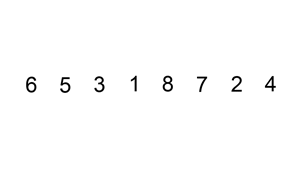# 插入排序的算法分析

2014/08/05 17:23

1. 从第一个元素开始，该元素可以认为已经被排序

2. 取出下一个元素，在已经排序的元素序列中从后向前扫描

3. 如果该元素（已排序）大于新元素，将该元素移到下一位置

4. 重复步骤3，直到找到已排序的元素小于或者等于新元素的位置

5. 将新元素插入到该位置后

6. 重复步骤2~5

# 插入排序使用C实现：

//插入排序
#include<stdio.h>
int main(){
int array={1,9,76,21,6,13,24,35};
int j;

/*
下标j指出正被插入到手中的当前牌。
在for循环（循环变量为j）每次迭代的开始
array[1..j-1]为当前已经排好序的牌，剩余的子数组array[j+1..n]对应于仍在桌子上的牌堆
*/

for(j=2;j<8;j++){
int key = array[j];
int i = j-1;
while(i>0 && array[i]>key){
array[i+1] = array[i];
i=i-1;
}
array[i+1] = key;
}

int n;
for(n = 0;n<8;n++){
printf("%d\n",array[n]);
}
}# 插入排序使用Java泛型实现

package aop;

public class InsertSort {

public static <T extends Comparable<? super T>> void sort(T[] array) {
for (int j = 2; j < array.length; j++) {
//insert array[j] into the sorted sequence
T key = array[j];
int i = j - 1;
while (i > 0 && array[i].compareTo(key) > 0) {
array[j] = array[i];
i--;
}
array[i + 1] = key;
}
}

public static void main(String args[]) {
String[] arrs = {"asd", "dsa", "cse", "sa", "eeww", "oiuu"};
System.out.println(arrs.length);
InsertSort.sort(arrs);
for (String s : arrs) {
System.out.println(s);
}
}
}

# 算法的渐近时间复杂度

====END====

0
1 收藏

### 作者的其它热门文章0 评论
1 收藏
0